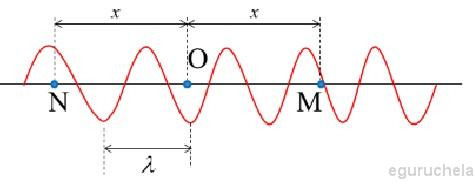﻿ All the formulas related to Periodic Motion

# List of formulas related to Periodic Motion

## Wavelength

$$\lambda = \frac{v}{f} = vT$$### Function Wave At Some Points

$$=> O : u_o = Acos \omega t$$ $$=> u_M = Acos[2 \pi(\frac{t}{T} - \frac{x}{\lambda})]$$ $$=> N : u_N = Acos[2 \pi(\frac{t}{T} + \frac{x}{\lambda})]$$

### Wave Interference

S1,S2: 2 sources of wave having same phase; M: a random point

$$\omega = \frac{v}{R}$$ $$\text{Function at point M}$$ $$u = 2A[cos \frac{\pi(d_2 - d_1)}{\lambda}] cos 2 \pi (ft - \frac{d_2 + d_1}{\lambda})$$ $$\text{Phase difference}$$ $$\Delta \phi = \frac{2 \pi}{\lambda}(d_1 - d_2)$$ $$\text{Constructive Points}$$ $$d_2 - d_1 = k \lambda$$ $$\text{Constructive Points}$$ $$d_2 - d_1 = (k + \frac{1}{2})\lambda$$